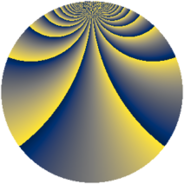# Properties

 Label 1792.2.jLevel $1792$ Weight $2$ Character orbit 1792.j Rep. character $\chi_{1792}(447,\cdot)$ Character field $\Q(\zeta_{4})$ Dimension $128$ Sturm bound $512$

# Learn more

## Defining parameters

 Level: $$N$$ $$=$$ $$1792 = 2^{8} \cdot 7$$ Weight: $$k$$ $$=$$ $$2$$ Character orbit: $$[\chi]$$ $$=$$ 1792.j (of order $$4$$ and degree $$2$$) Character conductor: $$\operatorname{cond}(\chi)$$ $$=$$ $$112$$ Character field: $$\Q(i)$$ Sturm bound: $$512$$

## Dimensions

The following table gives the dimensions of various subspaces of $$M_{2}(1792, [\chi])$$.

Total New Old
Modular forms 560 128 432
Cusp forms 464 128 336
Eisenstein series 96 0 96

## Trace form

 $$128q + O(q^{10})$$ $$128q - 128q^{81} + O(q^{100})$$

## Decomposition of $$S_{2}^{\mathrm{new}}(1792, [\chi])$$ into newform subspaces

The newforms in this space have not yet been added to the LMFDB.

## Decomposition of $$S_{2}^{\mathrm{old}}(1792, [\chi])$$ into lower level spaces

$$S_{2}^{\mathrm{old}}(1792, [\chi]) \cong$$ $$S_{2}^{\mathrm{new}}(112, [\chi])$$$$^{\oplus 5}$$$$\oplus$$$$S_{2}^{\mathrm{new}}(448, [\chi])$$$$^{\oplus 3}$$$$\oplus$$$$S_{2}^{\mathrm{new}}(896, [\chi])$$$$^{\oplus 2}$$